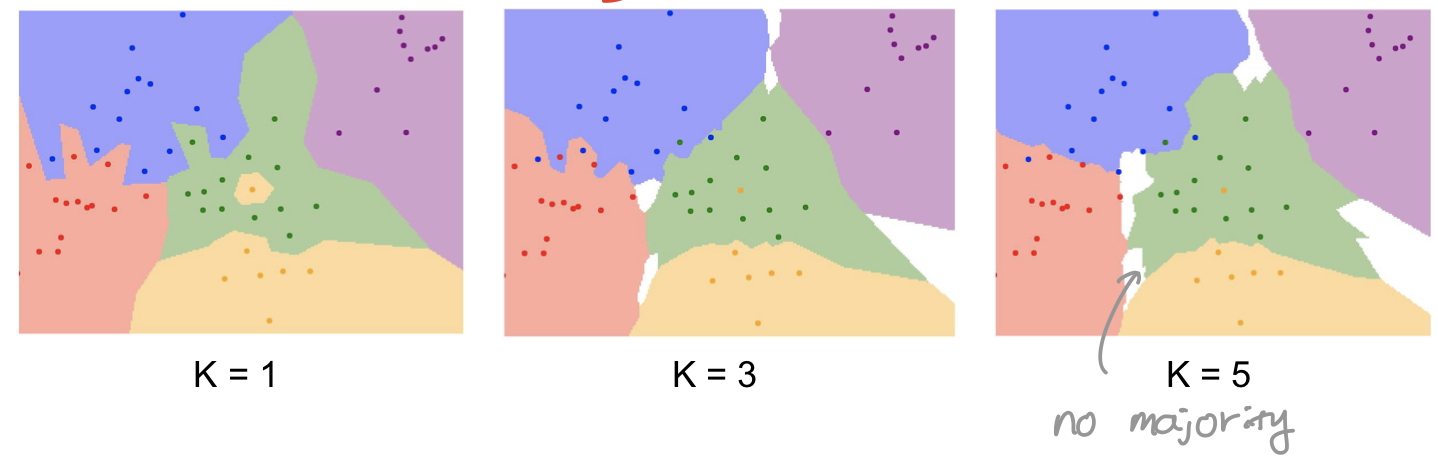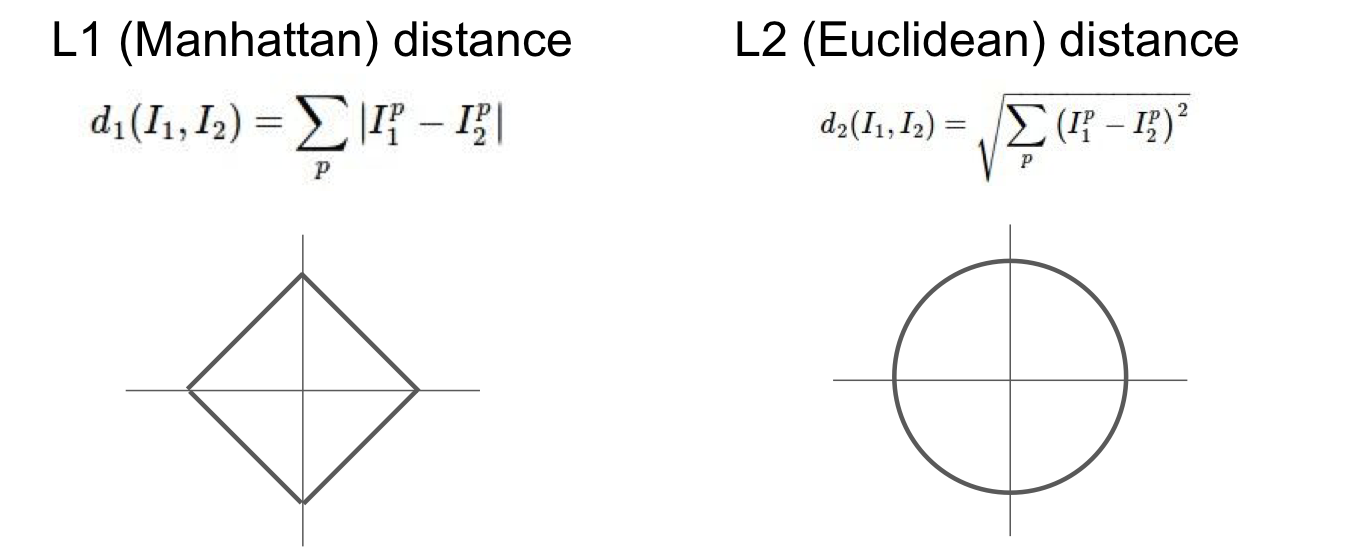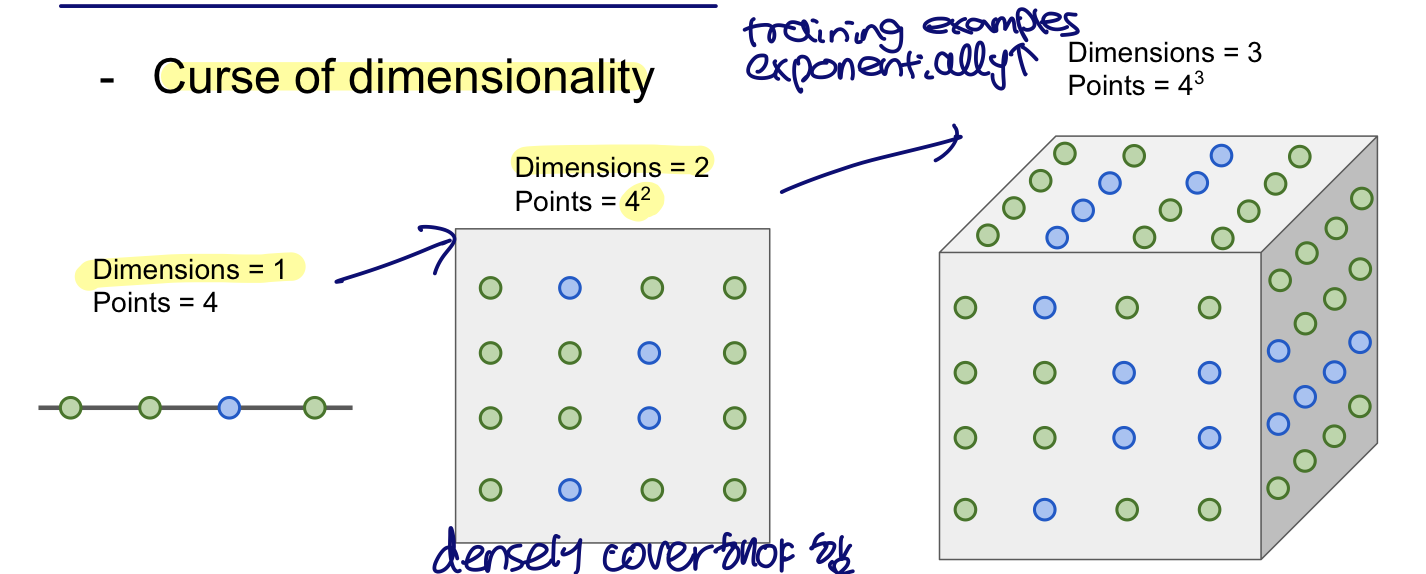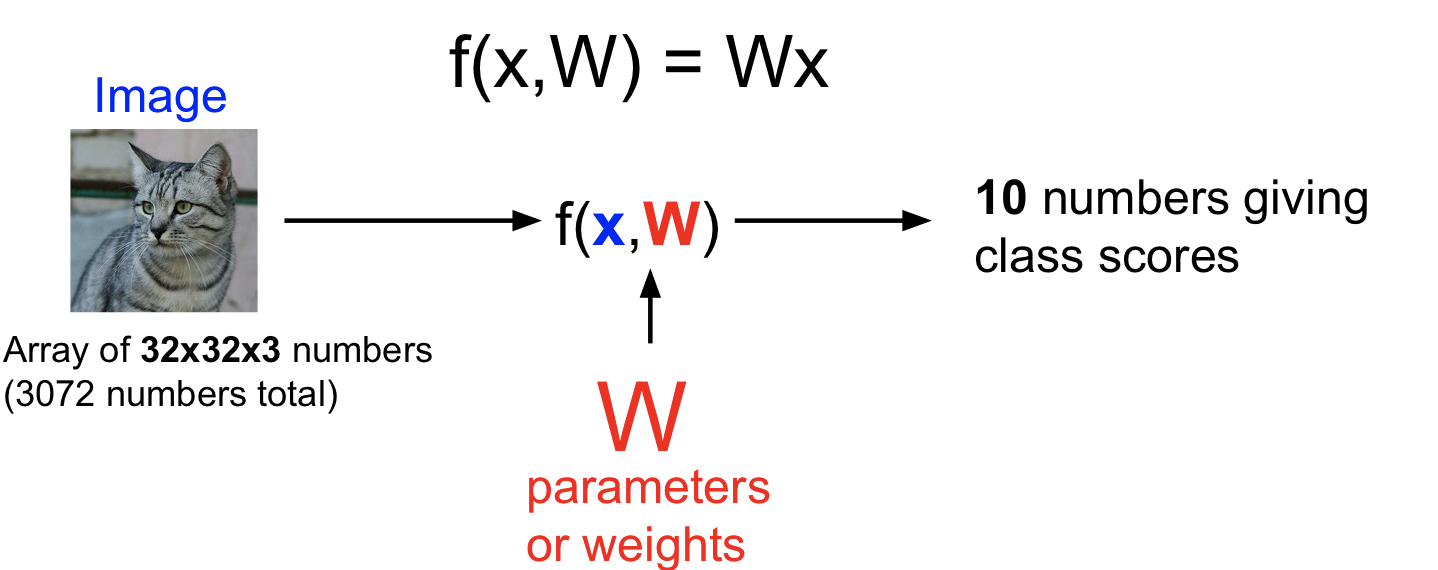Stanford CS231n 2017 강의를 듣고 개인적으로 정리한 글입니다.

# Lecture 2 : Image Classification pipeline

## Image Classification

• Computer Vision Task
• Problem is …
• Semantic Gap : between image and pixels (what the computer sees)
• Computer understands the image as a big grid of numbers (800,600,3)
• Challenges (algorithm should be robust to these challenges)
• Viewpoint variation
• all pixels change when the viewpoint is changed
• Illumination
• different light condition
• Deformation
• Example : cat…
• Occlusion
• The image shows just “part” of a cat
• Background Cluttuer
• I track as Variation

## Image Classifier

 123 def classify_image(image): # some magic! return class_label 
• Attempts have been made
• find edges and corners
• Data-Driven Approach
• Collect a dataset of images and labels
• Use ML to train a classifier
• Evaluate the classifier on new images

## First Classifier : Nearest Neighbor

• train : memorize all training data
• O(1)
• Predict : predict the label of most similar training image
• O(N)
• But we want classifier that are fast at prediction; slow for training is OK.**
• K-Nearest Nighbors
• Take majority vote from K closest points• Distance Metric (to compare images)• L1 distance(Manhattan distance)
• Calculate the difference of image
• Depends on choice of coordinate system
• Use when individual vector is meaningful
• L2 distance(Euclidean distance)
• Use when generic vector in some space
• kNN on images never used
• Very slow at test time
• Distance metrics on pixels are not informative
• couldn’t reflected “perceptional distance”
• Curse of dimensionality• If dimensions are increased in image, data points must densely cover to these dimensions
• Training examples are exponentially needed.

## Hyperparameters & Pipeline

• Problem-dependent
• try them all out and see what works best …
• Split data into train, val, and test
• underlying : the same probability distribution
• Cross-Validation
• Split data into folds
• We can know which hyperparameters are going to perform more robustly
• Useful to small datasets -> not used too frequently in deep learning

## Linear Classification

• Parametric Approach : summarize knowledge of training examples & stick all that knowledge into W.
• Image -> f(x,W) -> N numbers giving class scores
• W : parameters or weights
• f(x,W) = Wx + b (# of classes = 10, input dimension = 3072)
• f(x,W) : 10x1
• W should be 10 x 3072
• x : 3072x1
• b : 10x1
• Wx gives classes’ scores
• Bias
• constant vector
• Not interact with training set
• data independent, preferences for some classes
• Overview• Interpretation of linear classifiers as template matching
• 1 class : 1 template (driven from training data)
• Hard cases for a linear classifier
• Question
• how can we tell whether this W is good or bad?